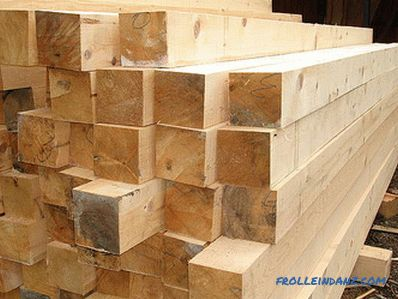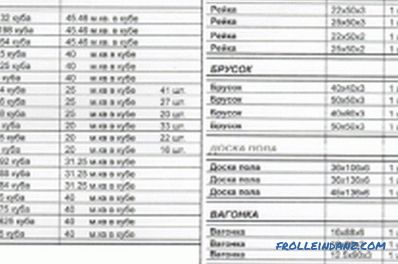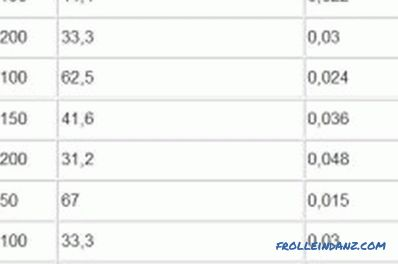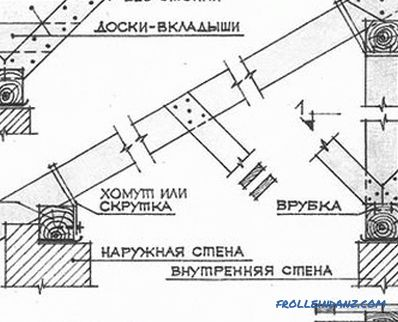# calculations and determination by tables (video)

Purchase of sawn timber is accompanied by calculations of their quantity. At the same time, most sellers voice the cost of wood in cubic meters, especially if the question concerns the type of materials whose elements do not have exactly the same size. Consider how you can calculate the number of boards when you buy one cube of edged material.The edging board is an important material in construction. Its required amount is measured in cubic meters.

## Calculation of edged boards

The trimmed timber represents sawn timber with treated surfaces. Gauges cut wood are in the range from 16 to 75 (thickness) and from 75 to 275 mm (width). The length can correspond to 3, 4 or 6 m.

To determine how many edged boards in the cube, you can use the simplest mathematical calculations.

Since edged lumber has the same geometry, their number in a cube can be determined by calculating the volume of one product unit. It is equal to the product of its three dimensions: width, thickness and length.

For correct calculations, all measured values ​​must be converted to meters. The resulting value will have the form of a decimal fraction and will be a numerical characteristic of the volume of a commodity unit.

Since the product being purchased has the same width, thickness and length, to determine its number, it is necessary to divide 1 m 3 by the received volume of the product unit.

Let us give an example of calculating the number of boards in a cube.Table for calculating the cubic capacity of sawn timber: edged boards, unedged boards, slats, bar, lining boards and floor boards.

Suppose we buy lumber 25 x 100 mm with a standard length of 6 m.

1. For calculations, we translate these values ​​into meters (using the formula 1 m = 1000 mm). That is, for translation, we divide the indicated parameters by 1000 (we move the comma to the left by three characters).

25 mm = 0.025 m

100 mm = 0.1 m

1. Perform multiplication using the formula V (volume) = width x thickness x length : 0.1 mx 0.025 mx 6 m = 0.015 m 3
2. We determine the number of edged boards in one cubic meter. To do this, divide 1 by the resulting value of the volume.

1 m / 0.015 m = 66.66 pieces.

Since whole lumber is supplied to you, the cost of 66 pieces will be slightly less than one cube of wood. However, often at sales the distributors assume that only 66 pieces of 25x100 (6 m in length) fit in one cube. What are such approximate calculations based on not in favor of the buyer?

## Determining the number of edged boards using the tables

For those who do not want to make calculations, tables have been developed (Fig. 1).

In the tables, the given values ​​are obtained by calculations. In some tables, the values ​​are rounded, sometimes the sellers themselves produce this rounding. Thus, according to rounded data, the number of edged material (with dimensions of 25 x 100, 6 m) may be 66 pieces (and not 66.66 units of goods obtained in the calculations).

Lack of volume and a small overpayment are sometimes compensated for by the fact that the size of the material exceeds the stipulated 6 meters (6.1 or 6.2 m). Such compensation is irrelevant when a designated length of 6 m is required in construction and an extra pair of centimeters does not matter.Figure 1. Determining the number of cut boards.

When purchasing several cubes of a tree, the missing 0.66 units of goods are added up to some additional quantity that the buyer must receive from the seller. Let's give an example.

Let us assume that a customer purchases 5 cubic meters of sawn timber at a store or on the market (cross section 25 x 100, 6 m). According to the table data often used by wood vendors, the buyer should receive 66 x 5 = 330 boards. In fact, the amount of the paid goods is 66.66 x 5 = 333. 3 pieces. That is, the seller must add at least three floorboards to the quantity determined by the table.

A buyer purchasing building materials must perform his own mathematical calculations to check the accuracy of the seller’s calculations. Correct calculations do honor to the supplier of building materials and cause respect to all categories of buyers, from individuals to large developers.

The existing mathematical calculations are based on the simplest formulas studied in the elementary school mathematics section.

Any educated person can cope with them. The use of calculators facilitates calculations and makes them quick, easy and accessible.

For adults who cannot cope with performing these mathematical operations, it is recommended to contact your own child for help. If your son or daughter is 10-11 years old, he will quite successfully cope with the set mathematical task.

•